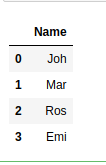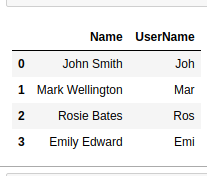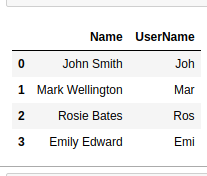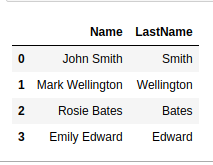# Get the substring of the column in Pandas-Python

Now, we’ll see how we can get the substring for all the values of a column in a Pandas dataframe. This extraction can be very useful when working with data. For example, we have the first name and last name of different people in a column and we need to extract the first 3 letters of their name to create their username.

Example 1:
We can loop through the range of the column and calculate the substring for each value in the column.

 `# importing pandas as pd ` `import` `pandas as pd  ` ` `  `# creating a dictionary ` `dict` `=` `{``'Name'``:[``"John Smith"``, ``"Mark Wellington"``,  ` `                ``"Rosie Bates"``, ``"Emily Edward"``]} ` ` `  `# converting the dictionary to a ` `# dataframe ` `df ``=` `pd.DataFrame.from_dict(``dict``) ` ` `  `# storing first 3 letters of name ` `for` `i ``in` `range``(``0``, ``len``(df)): ` `    ``df.iloc[i].Name ``=` `df.iloc[i].Name[:``3``] ` ` `  `df `

Output:Example 2: In this example we’ll use `str.slice()`.

 `# importing pandas as pd ` `import` `pandas as pd  ` ` `  `# creating a dictionary ` `dict` `=` `{``'Name'``:[``"John Smith"``, ``"Mark Wellington"``, ` `                ``"Rosie Bates"``, ``"Emily Edward"``]} ` ` `  `# converting the dictionary to a  ` `# dataframe ` `df ``=` `pd.DataFrame.from_dict(``dict``) ` ` `  `# storing first 3 letters of name as username ` `df[``'UserName'``] ``=` `df[``'Name'``].``str``.``slice``(``0``, ``3``) ` ` `  `df `

Output:Example 3: We can also use the str accessor in a different way by using square brackets.

 `# importing pandas as pd ` `import` `pandas as pd  ` ` `  `# creating a dictionary ` `dict` `=` `{``'Name'``:[``"John Smith"``, ``"Mark Wellington"``,  ` `                ``"Rosie Bates"``, ``"Emily Edward"``]} ` ` `  `# converting the dictionary to a dataframe ` `df ``=` `pd.DataFrame.from_dict(``dict``) ` ` `  `# storing first 3 letters of name as username ` `df[``'UserName'``] ``=` `df[``'Name'``].``str``[:``3``] ` ` `  `df `

Output:Example 4: We can also use str.extract for this task. In this example we’ll store last name of each person in “LastName” column.

 `# importing pandas as pd ` `import` `pandas as pd  ` ` `  `# creating a dictionary ` `dict` `=` `{``'Name'``:[``"John Smith"``, ``"Mark Wellington"``, ` `                ``"Rosie Bates"``, ``"Emily Edward"``]} ` ` `  `# converting the dictionary to a dataframe ` `df ``=` `pd.DataFrame.from_dict(``dict``) ` ` `  `# storing lastname of each person ` `df[``'LastName'``] ``=` `df.Name.``str``.extract(r``'\b(\w+)\$'``,  ` `                                     ``expand ``=` `True``) ` ` `  `df `

Output:My Personal Notes arrow_drop_upCheck out this Author's contributed articles.

If you like GeeksforGeeks and would like to contribute, you can also write an article using contribute.geeksforgeeks.org or mail your article to contribute@geeksforgeeks.org. See your article appearing on the GeeksforGeeks main page and help other Geeks.

Please Improve this article if you find anything incorrect by clicking on the "Improve Article" button below.

Article Tags :

Be the First to upvote.

Please write to us at contribute@geeksforgeeks.org to report any issue with the above content.